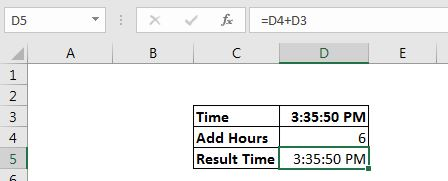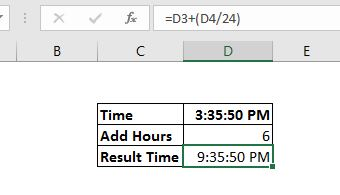# How to add Decimal Hours to Time

As we know that the date and time in excel are nothing but numbers. These numbers are formatted in such a way that they tell the exact time and date.

The problem arises when we try to add numeric or decimal time to a time formatted value. You can't just add them using the sum operator or function. Here's how you add decimal hours to time.

The generic formula for adding decimal hours:

 =Time+ (Hours/24)

or

 =Time+TIME(hours,0,0)

Time: It is the time to which you want to add hours.

Hours: Number of hours you want to add.

Let's see an example:In the snapshot above, we are trying to add 6 hours to time 3:35 PM, it should be 9:35 but it is not returning so. 1 hour in excel is equal to 1/24. It is explained here in detail. So we use the generic formulas mentioned above and try again.

The formula will be:

 =D3+(D4/24)

or

 =D3+TIME(D4,0,0)

This will return the correct time.How does it work?

As I said time in excel is not time but numbers. 1 day is equal to 1, 1 hour is equal to 1/24, 1 minute is equal to 1/24/60, and so on. I have explained the time and date in excel in detail here.

So when we want to add 6 decimal number and we directly add 6, it adds 6 days to the time. But when we add 6/24, it adds 6 hours to the time.

When we use the TIME function for adding hours in excel, the TIME function takes the first number and converts to hours and the sum operator sums the time to a given time.

This is it. Now you know how you can add decimal hours to a given time and get the correct resultant time. I hope it was explanatory. If you have any doubts regarding this article or any other excel/VBA related topic, ask me in the comments section below.

Related Articles

Time difference in hours as a decimal value | In simple words, We need to get the time difference in hours using Excel MOD function and then we need to represent it in the decimal format. MOD function returns the remainder when the number gets divided by a divisor.

How to Add Years to a Date |1 is equal to 1 day (24 hours). If you add multiples of 365 to a given date then it will give an incorrect answer, since there can be a leap year in between. To add years to a date in excel, we use a formula.

How to Add Months to a DateFor this example, I have this data. In cell A2, there is a start date, in B2 number of months to add and in C2 we will write the formula for adding months to this date.

How to Add Business Days in Excel | Excel provides a WORKDAY function. This function can be used to add business days a day. It excludes Saturdays, Sundays and optional holidays from the calculation of business days.

Popular Articles:

50 Excel Shortcuts to Increase Your Productivity | Get faster at your task. These 50 shortcuts will make you work even faster on Excel.

How to use Excel VLOOKUP Function| This is one of the most used and popular functions of excel that is used to lookup value from different ranges and sheets.

How to use the Excel COUNTIF Function| Count values with conditions using this amazing function. You don't need to filter your data to count specific value. Countif function is essential to prepare your dashboard.

How to Use SUMIF Function in Excel | This is another dashboard essential function. This helps you sum up values on specific conditions.

Terms and Conditions of use

The applications/code on this site are distributed as is and without warranties or liability. In no event shall the owner of the copyrights, or the authors of the applications/code be liable for any loss of profit, any problems or any damage resulting from the use or evaluation of the applications/code.